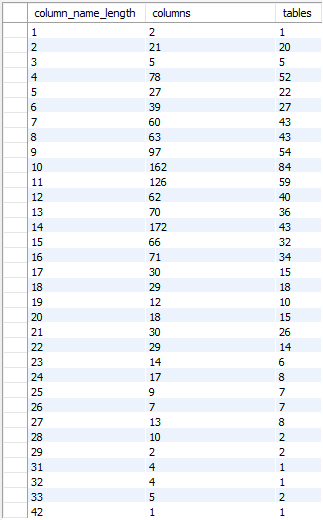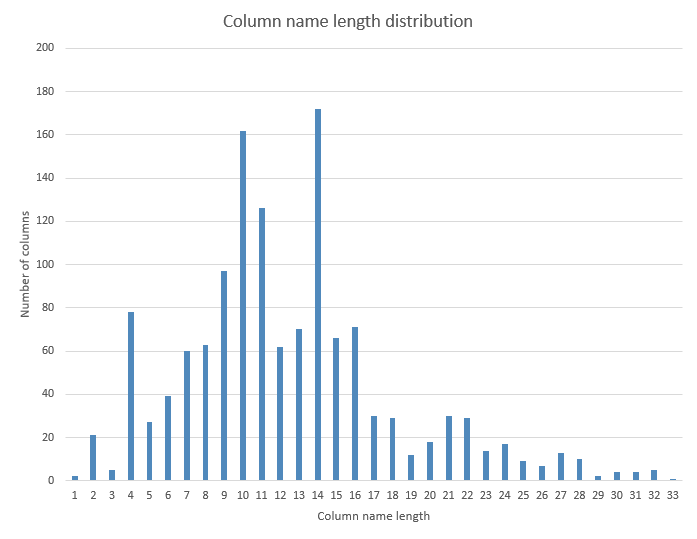## Get column name length distribution in MySQL database

Article for:

The query below returns the distribution of the column name lengths (number of characters) for all databases (schemas).

## Query

``````select char_length(col.column_name) as column_name_length,
count(*) as columns,
count(distinct tab.table_name) as tables
from information_schema.tables as tab
inner join information_schema.columns as col
on tab.table_schema = col.table_schema
and tab.table_name = col.table_name
where tab.table_type = 'BASE TABLE'
-- and tab.table_schema = 'your database name'
group by char_length(col.column_name)
order by char_length(col.column_name);
``````

Note: if you need the information for a specific database (schema), then uncomment the table_schema line and provide your database name.

## Columns

• column_name_length - length in column name characters
• columns - number of columns with this length
• tables - number of tables that have columns with this name length

## Rows

• One row: represents one name length (number of characters)
• Scope of rows: each column length that exists in a database (schema)
• Ordered by: ascending length (from 1 to max)

## Sample resultsIf you put this information in a chart, it will look like this: